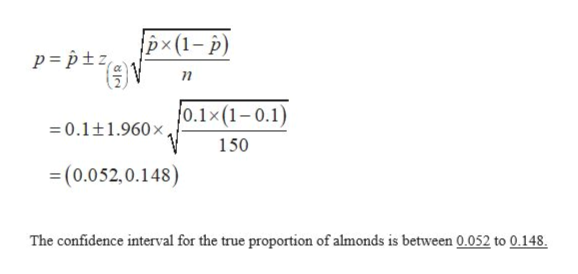# n a sample of 150 Planter’s Mixed Nuts, 15 were found to be almonds. (a) Construct a 95 percent confidence interval for the true proportion of almonds. (Use a z-value taken to three decimal places in your calculations. Round your answers to 3 decimal places.) The 95% confidence interval is from _________ to ________ . (b) May normality be assumed? YesNo  (c) What sample size would be needed for 95 percent confidence and an error of ±0.03? Sample size     _________

Question
60 views

n a sample of 150 Planter’s Mixed Nuts, 15 were found to be almonds.

(a) Construct a 95 percent confidence interval for the true proportion of almonds. (Use a z-value taken to three decimal places in your calculations. Round your answers to 3 decimal places.)

The 95% confidence interval is from _________ to ________ .

(b) May normality be assumed?

• Yes
• No

(c) What sample size would be needed for 95 percent confidence and an error of ±0.03?

Sample size     _________

check_circle

Step 1

According to the provided details, the sample of 150 Planter’s Mixed Nuts has been taken out of which 15 are almonds.

Therefore,

Sample size (n) = 150

Sample proportion () = 0.1.

The formula to calculate the 95% confidence interval for population proportion is:

Step 2

(a)

The critical value for 95% confidence level can be found using the z-table. That is,

Step 3

Substitute the values in the confi...help_outlineImage Transcriptionclosepx(1- p) p= p+z =0.1+1.960x0.1x(1-0.1) 150 (0.052,0.148) The confidence interval for the true proportion of almonds is between 0.052 to 0.148. fullscreen

### Want to see the full answer?

See Solution

#### Want to see this answer and more?

Solutions are written by subject experts who are available 24/7. Questions are typically answered within 1 hour.*

See Solution
*Response times may vary by subject and question.
Tagged in

### Other